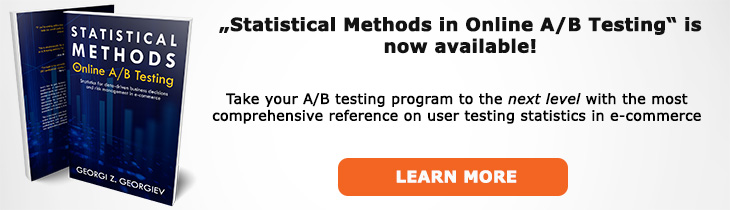# What does "Non-Parametric Test" mean?

Definition of Non-Parametric Test in the context of A/B testing (online controlled experiments).

## What is a Non-Parametric Test?

A non-parametric test is a statistical test that uses a non-parametric statistical model. Such a model makes fewer assumptions than a parametric one regarding the distribution of the parameter of interest (thus a more proper name is "low-parametric"). The main argument in favor of using non-parametric tests is that the practitioner does not commit to as many assumptions and so the model is less susceptible to violations of assumptions, e.g. a heavy-tailed distribution. This, however, is not necessarily the case as discussed below. The results from non-parametric tests are also harder to interpret due to their lower informational content.

The logic behind the claim that fewer assumptions makes for a more robust test (robust to violations of its premises) is that by allowing for the possibility of more distributions to describe the data, the likelihood that the set of allowable distribution contains the actual distribution is higher versus that of a parametric test which only allows one distribution. However, this presupposes that departures from the distribution assumptions of a test have the most severe consequences while in fact departures in other premises such as being identically distributed and independent are much more dangerous.

A result of the robustness of non-parametric models is that the results are less informative than those from parametric models, thus any conclusions made are less precise. Given that vague assumptions provide vague results due as one forgoes the optimality properties of parametric test and relies on asymptotic results and inequalities which may be wildly off-mark it is unclear if the relative freedom from assumptions is a preferable choice.

Tests based on non-parametric models usually suffer from a performance penalty in terms of power: they usually have less statistical power versus an applicable parametric test. The penalty is small, in most cases but the issue is in the asymptotic nature of the guarantees versus the finite-sample guarantees offered by parametric methods.

An example of a non-parametric test proposed for use in A/B testing is the Mann-Whitney-Wilcoxon rank-sum, particularly with regards to average revenue per user data. However, since the tested statistic is a mean following the central limit theorem its distribution is normal and thus the MWW R-S test is not needed. Furthermore, the MWW test is in fact testing the stochastic difference between the two data sets but how a practitioner is to interpret and make use of this data in practice is an example of how vague inferences can be a too high cost to pay for the relaxed assumptions.

## Related A/B Testing terms

Parametric Test

Statistical Model

Distribution

Like this glossary entry? For an in-depth and comprehensive reading on A/B testing stats, check out the book "Statistical Methods in Online A/B Testing" by the author of this glossary, Georgi Georgiev.## Glossary Index by Letter

Select a letter to see all A/B testing terms starting with that letter or visit the Glossary homepage to see all.